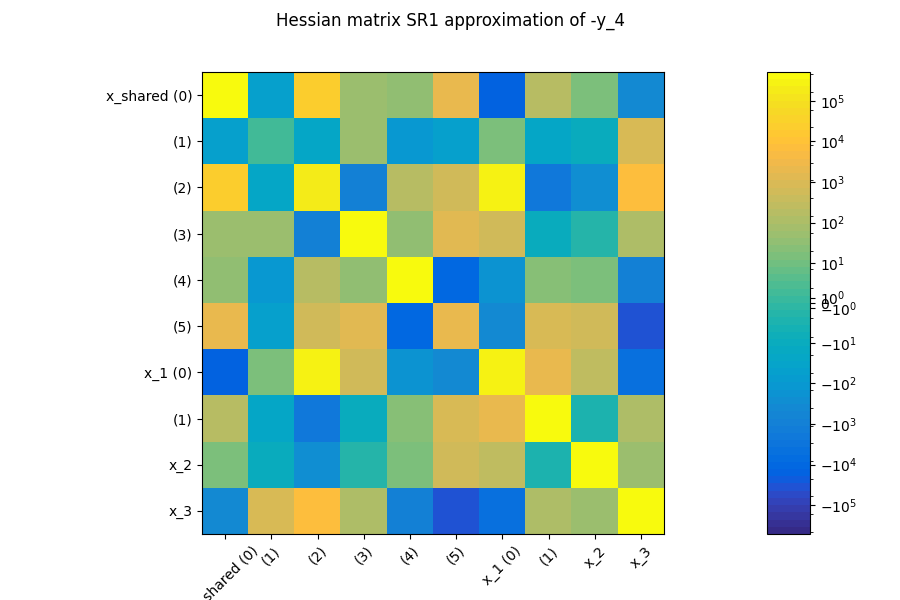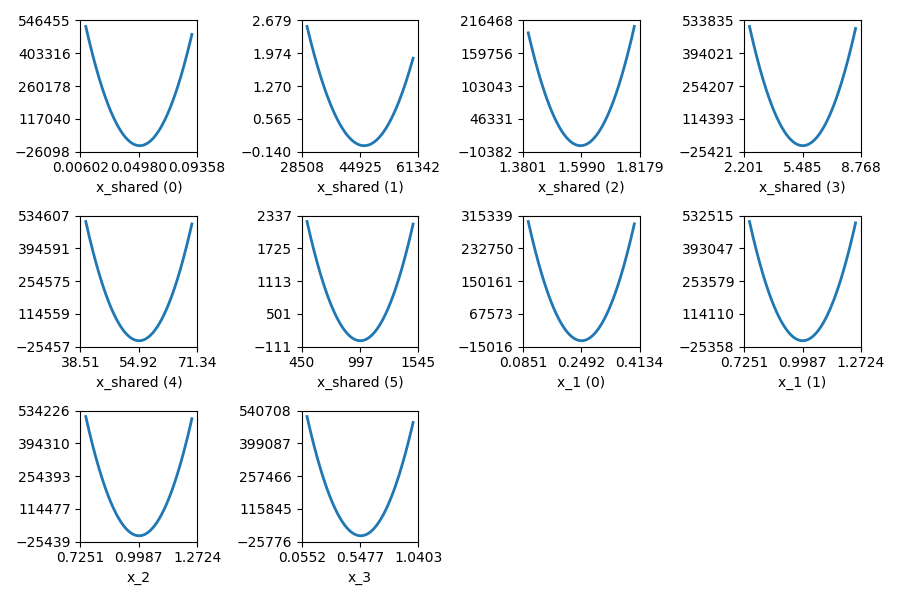In this example, we illustrate the use of the QuadApprox plot on the Sobieski’s SSBJ problem.

from __future__ import annotations

from gemseo.api import configure_logger
from gemseo.api import create_discipline
from gemseo.api import create_scenario
from gemseo.problems.sobieski.core.problem import SobieskiProblem


## Import¶

The first step is to import some functions from the API and a method to get the design space.

configure_logger()

<RootLogger root (INFO)>


## Description¶

The QuadApprox post-processing performs a quadratic approximation of a given function from an optimization history and plot the results as cuts of the approximation.

## Create disciplines¶

Then, we instantiate the disciplines of the Sobieski’s SSBJ problem: Propulsion, Aerodynamics, Structure and Mission

disciplines = create_discipline(
[
"SobieskiPropulsion",
"SobieskiAerodynamics",
"SobieskiStructure",
"SobieskiMission",
]
)


## Create design space¶

We also read the design space from the SobieskiProblem.

design_space = SobieskiProblem().design_space


## Create and execute scenario¶

The next step is to build an MDO scenario in order to maximize the range, encoded ‘y_4’, with respect to the design parameters, while satisfying the inequality constraints ‘g_1’, ‘g_2’ and ‘g_3’. We can use the MDF formulation, the SLSQP optimization algorithm and a maximum number of iterations equal to 100.

scenario = create_scenario(
disciplines,
formulation="MDF",
objective_name="y_4",
maximize_objective=True,
design_space=design_space,
)
scenario.set_differentiation_method()
for constraint in ["g_1", "g_2", "g_3"]:
scenario.execute({"algo": "SLSQP", "max_iter": 10})

    INFO - 16:55:34:
INFO - 16:55:34: *** Start MDOScenario execution ***
INFO - 16:55:34: MDOScenario
INFO - 16:55:34:    Disciplines: SobieskiAerodynamics SobieskiMission SobieskiPropulsion SobieskiStructure
INFO - 16:55:34:    MDO formulation: MDF
INFO - 16:55:34: Optimization problem:
INFO - 16:55:34:    minimize -y_4(x_shared, x_1, x_2, x_3) = -y_4(x_shared, x_1, x_2, x_3)
INFO - 16:55:34:    with respect to x_1, x_2, x_3, x_shared
INFO - 16:55:34:    subject to constraints:
INFO - 16:55:34:       g_1(x_shared, x_1, x_2, x_3) <= 0.0
INFO - 16:55:34:       g_2(x_shared, x_1, x_2, x_3) <= 0.0
INFO - 16:55:34:       g_3(x_shared, x_1, x_2, x_3) <= 0.0
INFO - 16:55:34:    over the design space:
INFO - 16:55:34:    +-------------+-------------+-------+-------------+-------+
INFO - 16:55:34:    | name        | lower_bound | value | upper_bound | type  |
INFO - 16:55:34:    +-------------+-------------+-------+-------------+-------+
INFO - 16:55:34:    | x_shared |     0.01    |  0.05 |     0.09    | float |
INFO - 16:55:34:    | x_shared |    30000    | 45000 |    60000    | float |
INFO - 16:55:34:    | x_shared |     1.4     |  1.6  |     1.8     | float |
INFO - 16:55:34:    | x_shared |     2.5     |  5.5  |     8.5     | float |
INFO - 16:55:34:    | x_shared |      40     |   55  |      70     | float |
INFO - 16:55:34:    | x_shared |     500     |  1000 |     1500    | float |
INFO - 16:55:34:    | x_1      |     0.1     |  0.25 |     0.4     | float |
INFO - 16:55:34:    | x_1      |     0.75    |   1   |     1.25    | float |
INFO - 16:55:34:    | x_2         |     0.75    |   1   |     1.25    | float |
INFO - 16:55:34:    | x_3         |     0.1     |  0.5  |      1      | float |
INFO - 16:55:34:    +-------------+-------------+-------+-------------+-------+
INFO - 16:55:34: Solving optimization problem with algorithm SLSQP:
INFO - 16:55:34: ...   0%|          | 0/10 [00:00<?, ?it]
INFO - 16:55:34: ...  10%|█         | 1/10 [00:00<00:00, 11.40 it/sec, obj=-536]
INFO - 16:55:34: ...  20%|██        | 2/10 [00:00<00:00,  8.87 it/sec, obj=-2.12e+3]
WARNING - 16:55:34: MDAJacobi has reached its maximum number of iterations but the normed residual 1.4486313079508508e-06 is still above the tolerance 1e-06.
INFO - 16:55:34: ...  30%|███       | 3/10 [00:00<00:00,  7.69 it/sec, obj=-3.75e+3]
INFO - 16:55:34: ...  40%|████      | 4/10 [00:00<00:00,  7.51 it/sec, obj=-4.01e+3]
WARNING - 16:55:34: MDAJacobi has reached its maximum number of iterations but the normed residual 2.928004141058104e-06 is still above the tolerance 1e-06.
INFO - 16:55:34: ...  50%|█████     | 5/10 [00:00<00:00,  7.16 it/sec, obj=-4.49e+3]
INFO - 16:55:34: ...  60%|██████    | 6/10 [00:00<00:00,  7.29 it/sec, obj=-3.4e+3]
INFO - 16:55:34: ...  70%|███████   | 7/10 [00:00<00:00,  7.91 it/sec, obj=-4.93e+3]
INFO - 16:55:35: ...  80%|████████  | 8/10 [00:00<00:00,  8.25 it/sec, obj=-4.76e+3]
INFO - 16:55:35: ...  90%|█████████ | 9/10 [00:01<00:00,  8.58 it/sec, obj=-4.62e+3]
INFO - 16:55:35: ... 100%|██████████| 10/10 [00:01<00:00,  8.95 it/sec, obj=-4.56e+3]
INFO - 16:55:35: Optimization result:
INFO - 16:55:35:    Optimizer info:
INFO - 16:55:35:       Status: None
INFO - 16:55:35:       Message: Maximum number of iterations reached. GEMSEO Stopped the driver
INFO - 16:55:35:       Number of calls to the objective function by the optimizer: 12
INFO - 16:55:35:    Solution:
INFO - 16:55:35:       The solution is feasible.
INFO - 16:55:35:       Objective: -3749.8868975554387
INFO - 16:55:35:       Standardized constraints:
INFO - 16:55:35:          g_1 = [-0.01671296 -0.03238836 -0.04350867 -0.05123129 -0.05681738 -0.13780658
INFO - 16:55:35:  -0.10219342]
INFO - 16:55:35:          g_2 = -0.0004062839430756249
INFO - 16:55:35:          g_3 = [-0.66482546 -0.33517454 -0.11023156 -0.183255  ]
INFO - 16:55:35:       Design space:
INFO - 16:55:35:       +-------------+-------------+---------------------+-------------+-------+
INFO - 16:55:35:       | name        | lower_bound |        value        | upper_bound | type  |
INFO - 16:55:35:       +-------------+-------------+---------------------+-------------+-------+
INFO - 16:55:35:       | x_shared |     0.01    | 0.05989842901423112 |     0.09    | float |
INFO - 16:55:35:       | x_shared |    30000    |  59853.73840058666  |    60000    | float |
INFO - 16:55:35:       | x_shared |     1.4     |         1.4         |     1.8     | float |
INFO - 16:55:35:       | x_shared |     2.5     |  2.527371250092273  |     8.5     | float |
INFO - 16:55:35:       | x_shared |      40     |  69.86825198198687  |      70     | float |
INFO - 16:55:35:       | x_shared |     500     |  1495.734648986894  |     1500    | float |
INFO - 16:55:35:       | x_1      |     0.1     |         0.4         |     0.4     | float |
INFO - 16:55:35:       | x_1      |     0.75    |  0.7521124139939552 |     1.25    | float |
INFO - 16:55:35:       | x_2         |     0.75    |  0.7520888531444992 |     1.25    | float |
INFO - 16:55:35:       | x_3         |     0.1     |  0.1398000762238233 |      1      | float |
INFO - 16:55:35:       +-------------+-------------+---------------------+-------------+-------+
INFO - 16:55:35: *** End MDOScenario execution (time: 0:00:01.134528) ***

{'max_iter': 10, 'algo': 'SLSQP'}


## Post-process scenario¶

Lastly, we post-process the scenario by means of the QuadApprox plot which performs a quadratic approximation of a given function from an optimization history and plot the results as cuts of the approximation.

Tip

Each post-processing method requires different inputs and offers a variety of customization options. Use the API function get_post_processing_options_schema() to print a table with the options for any post-processing algorithm. Or refer to our dedicated page: Post-processing algorithms.

The first plot shows an approximation of the Hessian matrix $$\frac{\partial^2 f}{\partial x_i \partial x_j}$$ based on the Symmetric Rank 1 method (SR1) [NW06]. The color map uses a symmetric logarithmic (symlog) scale. This plots the cross influence of the design variables on the objective function or constraints. For instance, on the last figure, the maximal second-order sensitivity is $$\frac{\partial^2 -y_4}{\partial^2 x_0} = 2.10^5$$, which means that the $$x_0$$ is the most influential variable. Then, the cross derivative $$\frac{\partial^2 -y_4}{\partial x_0 \partial x_2} = 5.10^4$$ is positive and relatively high compared to the previous one but the combined effects of $$x_0$$ and $$x_2$$ are non-negligible in comparison.

scenario.post_process("QuadApprox", function="-y_4", save=False, show=True)

••<gemseo.post.quad_approx.QuadApprox object at 0x7fbc56179dc0>


The second plot represents the quadratic approximation of the objective around the optimal solution : $$a_{i}(t)=0.5 (t-x^*_i)^2 \frac{\partial^2 f}{\partial x_i^2} + (t-x^*_i) \frac{\partial f}{\partial x_i} + f(x^*)$$, where $$x^*$$ is the optimal solution. This approximation highlights the sensitivity of the objective function with respect to the design variables: we notice that the design variables $$x\_1, x\_5, x\_6$$ have little influence , whereas $$x\_0, x\_2, x\_9$$ have a huge influence on the objective. This trend is also noted in the diagonal terms of the Hessian matrix $$\frac{\partial^2 f}{\partial x_i^2}$$.

Total running time of the script: ( 0 minutes 1.896 seconds)

Gallery generated by Sphinx-Gallery